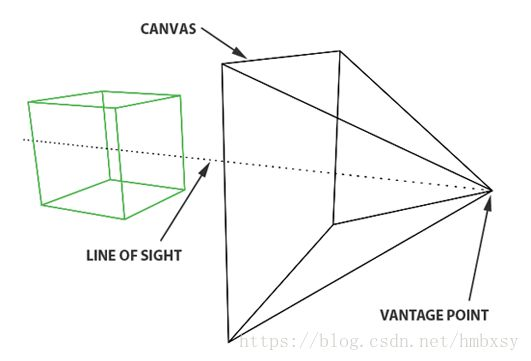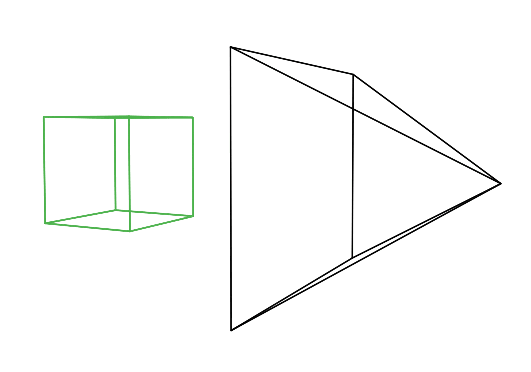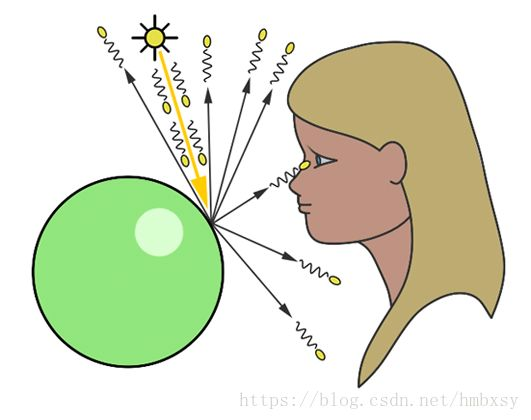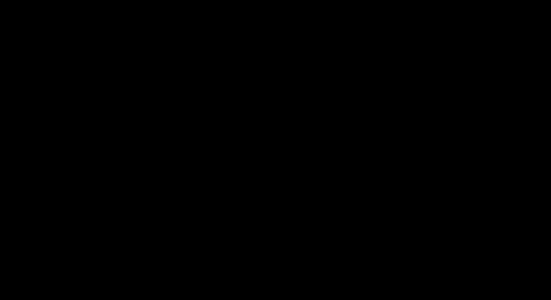# 光线追踪算法综述，路径追踪与GI技术进化编年史

## Problem Formulation

Ray
Tracing的目标是生成一张包含场景内物体，具有真实感的图像，因此实现一个简单的Ray
Tracing算法并不需要显式地构建一个可视的三维场景，只需要隐式地构建三维空间就可以了（也就是说这个三维场景只要存在你的脑袋里就可以了）。生成包含酒杯的渲染图像并不是一件很简单的事情，但是只生成包含几个简单几何体的渲染图，只需要两三百行代码。不需要图形库函数，只需要最基本的STL库。

Ray
Tracing能够实现一些使得画面更具真实感的效果，包括阴影、折射和反射，这些效果的本质是这张图片中颜色的变化，接下来我们将讨论如何量化这些效果，也就是量化这些颜色的变化。为了这些效果，我们也要对object的性质量化：表面颜色，反射性质，透射性质，然后利用公式计算得到每一个像素点的颜色。

## Basic Knowledge

Fresnel定律：

F=f_0+(1-f_0)(1-V*H)^5

f_0是入射角度接近0时的反射系数，V是指向视点的观察方向，H是半角向量。随着入射角趋近于直角，反射系数趋近1，则所有入射光都会被反射。F是反射系数，将用于折射光线和反射光线产生的效果的混合。

1、三维场景中创建图像

## 程序结构

cl -o a.exe RayTracer.cpp
a.exe

g++ -o a RayTracer.cpp
./a

color，用于记录这个像素的颜色。我们可以通过向量加减乘除，简单计算出和球体的交点，交点的法线方向（当然不同的几何体法线计算不同，你可以考虑自己在物体类内实现一个法线计算函数）。记得判断一下射线起点是否在第一个交点所在物体内，进行一下符号变换，这个在折射光线计算的时候很重要。

Effect，它和入射光线方向和法线方向有关，这里我们用三次方来算，为了更快。f0=0.1和物体的材质有关系。

float facingratio = - raydirection.dot(nhit);
float fresneleffect = mix (pow(1 - facingratio, 3), 1, 0.1);
float mix(const float &a, const float &b, const float &mix)
{
return b * mix + a * (1 - mix);
}


$$n_isin\theta_i=n_Tsin\theta_T cos\theta_T=\sqrt{1-\frac{n_i2(1-cos2\theta_i)}{n^2_T}}IN=cos\theta_i|I||N| (-N) T=cos\theta_T|N||T| T=-\frac{n_i}{n_T}I+(\frac{n_i}{n_T}(IN)-cos\theta_T)*N$$
（这部分是Latex公式，在简书中支持不是很好，可以直接查看夏老师的PPT）

color。

surfaceColor = (next_reflection * fresneleffect + next_refraction * (1 - fresneleffect) * sphere->transparency) * sphere->surfaceColor;


    surfaceColor += sphere->surfaceColor * transmission * std::max(float(0), nhit.dot(lightDirection)) * spheres[i].emissionColor;


Render程序：此时就要生成一个图像了，因此我们的输入是整个场景，我们从起点到每一个像素坐标做一条射线，然后执行trace程序，返回这个像素的颜色信息，最后保存成图片格式就可以了。

## 关键结构

function来计算反射和折射的综合效果。

## 程序展示

x, y, z轴的右手守则

Vector Class：定义各种向量运算。

Light Source
Class：可以看成球体的一个特例。但是如果不加光源，只会是黑色的。

Object
Class：定义Object的运动，旋转、平移，和某一射线的交点（根据不同的形状）。如果想做视频的话，你可以考虑定义物体的运动或者视角的运动，这样你可以逐帧渲染，最后生成一个好的视频。

Mesh表示三维模型，这个时候我们计算到的交点的性质需要包含这个点的三角面片的三个顶点决定。

Trace Ray Function：just return a color。## 继续优化

1.构建一个Scene（包括背景颜色），向Scene中添加物体或者Set Union。

2.构建多样化的多面体，包括圆环、cube等。可以构造一个SetUnion作为允许装载多种object的容器。光线与平面、三角形、多边形、长方体等求交。

3.添加纹理效果（面片顶点指定纹理，对面片和光线的交点进行插值）。

4.加速算法：包围盒加速、层次结构加速。

• 全局光照的基本概念
• 全局光照的算法主要流派
• 全局光照技术进化编年史
• 光线追踪 Ray Tracing
• 路径追踪 Path Tracing
• 光线追踪、路径追踪、光线投射的区别
• 环境光遮蔽 Ambient Occlusion## 程序

//Compile using clang under Windows: cl -o RayTracer.exe RayTracer.cpp

#include <cstdlib>
#include <cstdio>
#include <cmath>
#include <fstream>
#include <vector>
#include <iostream>
#include <cassert>
#include <algorithm>

#define M_PI 3.141592653589
#define INFINITY 1e8

//define class Vec_3, used in ray direction
template<typename T>
class Vec_3
{
public:
T x, y, z;
Vec_3(): x(T(0)), y(T(0)), z(T(0)) {}
Vec_3(T xx): x(xx), y(xx), z(xx) {}
Vec_3(T xx, T yy, T zz): x(xx), y(yy), z(zz){}
Vec_3<T> operator * (const T &f) const
{ return Vec_3<T>(x * f, y * f, z * f);}
Vec_3<T> operator * (const Vec_3<T> &v) const
{ return Vec_3<T>(x * v.x, y * v.y, z * v.z);}
T dot(const Vec_3<T> &v) const
{ return x * v.x + y * v.y + z * v.z;}
Vec_3<T> operator - (const Vec_3<T> &v) const
{ return Vec_3<T>( x - v.x, y - v.y, z - v.z);}
Vec_3<T> operator + (const  Vec_3<T> &v) const
{ return Vec_3<T>( x + v.x, y + v.y, z + v.z);}
Vec_3<T>& operator += (const Vec_3<T> &v)
{
x += v.x;
y += v.y;
z += v.z;
return *this;
}
Vec_3<T>& operator *= (const Vec_3<T> &v)
{
x *= v.x;
y *= v.y;
z *= v.z;
return *this;
}
Vec_3<T> operator - () const
{
return Vec_3<T>(-x, -y, -z);
}
T length2() const
{
return x * x + y * y + z * z;
}
T length() const
{
return sqrt(length2());
}
Vec_3& normal()
{
T nor2= length2();
if (nor2 > 0)
{
T nor2_inv= 1 / sqrt(nor2);
x *= nor2_inv;
y *= nor2_inv;
z *= nor2_inv;
}
return *this;
}
friend std::ostream & operator << (std::ostream &os, const Vec_3<T> &v)
{
os<< "[" << v.x << " " << v.y << " " << v.z << "]";
return os;
}
};

typedef Vec_3<float> Vec_3f;

//Define Sphere Class
class Sphere
{
public:
Vec_3f center;
Vec_3f surfaceColor, emissionColor;
float transparency, reflection;
Sphere(
const Vec_3f &c,
const float &r,
const Vec_3f &sc,
const float &refl = 0,
const float &transp = 0,
const Vec_3f &ec = 0):
center(c), radius(r), radius2(r * r), surfaceColor(sc), emissionColor(ec),
transparency(transp), reflection(refl)
{}
//Use geometric solution to solve a ray-sphere intersection
bool intersect(const Vec_3f &rayorigin, const Vec_3f & raydirection, float &t0, float &t1) const
{
Vec_3f l = center - rayorigin;
//Determine whether reverse direction
float tca = l.dot(raydirection);
if  (tca < 0) return false;
//a^2=b^2+c^2
float dist = l.dot(l) - tca * tca;
if (dist > radius2) return false;
float thc = sqrt(radius2 - dist);
//t0: first intersection distance, t1: second intersection distance
t0 = tca - thc;
t1 = tca + thc;

return true;
}
};

//Define the maximum recursion depth
#define MAX_DEPTH 5

//Calculate the mix value for reflection and refraction
float mix(const float &a, const float &b, const float &mix)
{
return b * mix + a * (1 - mix);
}

//Ray Tracing Function: takes a ray (defined by its origin and direction) as argument.
//Through the function, we can know if the ray intersects any of the geometry in the scene.
//If the ray intersects an object, calculate the intersection point and its normal, then shade the point.
//Shading depends on the surface (transparent, reflective, diffuse)
//If the ray intersects an object, then return the color of the object at the intersection point, otherwise return the backgroud color.
Vec_3f trace(
const Vec_3f &rayorigin,
const Vec_3f &raydirection,
const std::vector<Sphere> &spheres,
const int &depth
)
{
float tnear= INFINITY;
const Sphere* sphere=NULL;
//calculate intersection of this ray with the sphere in the scene
for(unsigned i=0; i < spheres.size(); i++)
{
float t0=INFINITY;
float t1=INFINITY;
if(spheres[i].intersect(rayorigin, raydirection, t0, t1))
{
//If the point in the sphere
if(t0 < 0) t0= t1;
if(t0 < tnear)
{
tnear = t0;
sphere = &spheres[i];
}
}
}
//If there is no intersection, then return backgroud color
if(!sphere) return Vec_3f(0);
//Color of ray
Vec_3f surfaceColor = 0;
//point of intersect
Vec_3f phit = rayorigin + raydirection * tnear;
//normal of the intersection point
Vec_3f nhit = phit - sphere->center;
//normalize the normal direction
nhit.normal();
//If the normal and the view direction's dot is positive, means the view point inside sphere
float bias = 1e-4;
bool inside = false;
if(raydirection.dot(nhit) > 0)
{
nhit = -nhit;
inside = true;
}
//Tackle with relection and refraction
if((sphere->transparency > 0 || sphere->reflection > 0) && depth < MAX_DEPTH)
{
//Compute fresnel effect
float facingratio = - raydirection.dot(nhit);
float fresneleffect = mix (pow(1 - facingratio, 3), 1, 0.1);
//Compute reflection direction
Vec_3f reflect_direction = raydirection - nhit * 2 * raydirection.dot(nhit);
reflect_direction.normal();
Vec_3f next_reflection = trace(phit + nhit * bias, reflect_direction, spheres, depth + 1);
//Vec_3f next_reflection = trace(phit, reflect_direction, spheres, depth + 1);
Vec_3f next_refraction = 0;
//Only if the sphere is transparent, then compute refraction ray
if(sphere->transparency)
{
//judge whether we are inside or outside? ior is the index of two materials
float ior = 1.1, eta = (inside) ? ior : 1 / ior;
float cosi = -nhit.dot(raydirection);
float k = 1 - eta * eta * (1 - cosi * cosi);
Vec_3f refraction_direction = raydirection * eta + nhit * (eta * cosi - sqrt(k));
refraction_direction.normal();
next_refraction = trace(phit - nhit * bias, refraction_direction, spheres, depth+1);
//next_refraction = trace(phit, refraction_direction, spheres, depth+1);
}
//The surface is a mix of reflection and refraction (if the sphere is transparent)
surfaceColor = (next_reflection * fresneleffect + next_refraction * (1 - fresneleffect) * sphere->transparency) * sphere->surfaceColor;
}
//If it is a diffuse object, no need to ray tracing.
else
{
for(unsigned i = 0; i < spheres.size(); i++)
{
//This is a light
if(spheres[i].emissionColor.x > 0)
{
Vec_3f transmission = 1;
Vec_3f lightDirection = spheres[i].center - phit;
lightDirection.normal();
//Check whether have an obstacle between light and object, add shadow
for(unsigned j = 0; j < spheres.size(); ++j)
{
if(i != j)
{
float t0, t1;
if(spheres[j].intersect(phit + nhit * bias, lightDirection, t0, t1))
//if(spheres[j].intersect(phit, lightDirection, t0, t1))
{
transmission = 0;
break;
}
}
}

//If nhit and lightDirection's dot is less than 0, then no light.
surfaceColor += sphere->surfaceColor * transmission * std::max(float(0), nhit.dot(lightDirection)) * spheres[i].emissionColor;
}
}
}

return surfaceColor + sphere->emissionColor;

}

//Render function, compute each pixel of the image.
void render(const std::vector<Sphere> &spheres)
{
unsigned width = 640, height = 480;
Vec_3f *img = new Vec_3f[width * height], *pixel = img;
float invWidth = 1 / float(width), invHeight = 1 / float(height);
float fov = 30;
float aspectratio = width / float(height);
float angle = tan(M_PI * 0.5 * fov / 180.);
//Trace all ray
for(unsigned y = 0; y < height; y++)
{
for(unsigned x = 0; x < width; x++, pixel++)
{
float xx = (2 * ((x + 0.5) * invWidth) - 1) * angle * aspectratio;
float yy = (1 - 2 * ((y + 0.5) * invHeight)) * angle;
Vec_3f raydir(xx, yy, -1);
raydir.normal();
*pixel = trace(Vec_3f(0), raydir, spheres, 0);
}
}
//Save the result
std::ofstream ofs("./1.ppm", std::ios::out | std::ios::binary);
ofs << "P6\n" << width << " " << height << "\n255\n";
for(unsigned i = 0; i < width * height; i++)
{
//0,255
ofs << (unsigned char)(std::min(float(1), img[i].x) * 255) <<
(unsigned char)(std::min(float(1), img[i].y) * 255) <<
(unsigned char)(std::min(float(1), img[i].z) * 255);
}
ofs.close();
delete [] img;
}

//Create a sign including 5 spheres and 1 light (which is also a sphere), then render it.
int main()
{
std::vector<Sphere> spheres;
//argument: position, radius, surfaceColor, reflectivity, transparency, emissionColor
spheres.push_back(Sphere(Vec_3f( 0.0,      0, -20),     4, Vec_3f(1.00, 0.00, 0.00), 1, 0.5));
spheres.push_back(Sphere(Vec_3f( 5.0,     -1, -15),     2, Vec_3f(0.00, 1.00, 0.00), 1, 0.0));
spheres.push_back(Sphere(Vec_3f( 5.0,      0, -25),     3, Vec_3f(0.00, 0.00, 1.00), 1, 0.0));
spheres.push_back(Sphere(Vec_3f(-5.5,      0, -15),     3, Vec_3f(0.00, 1.00, 0.00), 1, 0.0));
//Light
spheres.push_back(Sphere(Vec_3f(0.0, 20, -30), 3, Vec_3f(0.0, 0.0, 0.0), 0, 0.0, Vec_3f(3)));
render(spheres);

return 0;
}


2、物体的颜色和亮度

## 结果1 (2).png1 (1).png1 (3).png1 (4).png1 (5).png

## 最短的光线追踪程序

#include <stdlib.h>   // card > aek.ppm
#include <stdio.h>
#include <math.h>
typedef int i;typedef float f;struct v{f x,y,z;v operator+(v r){return v(x+r.x,y+r.y,z+r.z);}v operator*(f r){return v(x*r,y*r,z*r);}f operator%(v r){return x*r.x+y*r.y+z*r.z;}v(){}v operator^(v r){return v(y*r.z-z*r.y,z*r.x-x*r.z,x*r.y-y*r.x);}v(f a,f b,f c){x=a;y=b;z=c;}v operator!(){return*this*(1/sqrt(*this%*this));}};i G[]={247570,280596,280600,249748,18578,18577,231184,16,16};f R(){return(f)rand()/RAND_MAX;}i T(v o,v d,f&t,v&n){t=1e9;i m=0;f p=-o.z/d.z;if(.01<p)t=p,n=v(0,0,1),m=1;for(i k=19;k--;)for(i j=9;j--;)if(G[j]&1<<k){v p=o+v(-k,0,-j-4);f b=p%d,c=p%p-1,q=b*b-c;if(q>0){f s=-b-sqrt(q);if(s<t&&s>.01)t=s,n=!(p+d*t),m=2;}}return m;}v S(v o,v d){f t;v n;i m=T(o,d,t,n);if(!m)return v(.7,.6,1)*pow(1-d.z,4);v h=o+d*t,l=!(v(9+R(),9+R(),16)+h*-1),r=d+n*(n%d*-2);f b=l%n;if(b<0||T(h,l,t,n))b=0;f p=pow(l%r*(b>0),99);if(m&1){h=h*.2;return((i)(ceil(h.x)+ceil(h.y))&1?v(3,1,1):v(3,3,3))*(b*.2+.1);}return v(p,p,p)+S(h,r)*.5;}i main(){printf("P6 512 512 255 ");v g=!v(-6,-16,0),a=!(v(0,0,1)^g)*.002,b=!(g^a)*.002,c=(a+b)*-256+g;for(i y=512;y--;)for(i x=512;x--;){v p(13,13,13);for(i r=64;r--;){v t=a*(R()-.5)*99+b*(R()-.5)*99;p=S(v(17,16,8)+t,!(t*-1+(a*(R()+x)+b*(y+R())+c)*16))*3.5+p;}printf("%c%c%c",(i)p.x,(i)p.y,(i)p.z);}}


## Reference

1. http://www.cosinekitty.com/raytrace/contents.html
2. http://www.scratchapixel.com/

## 一、行文思路说明

Rendering 2nd》中，其实是放在Chapter 6 Advanced Lighting and

3rd》第九章标题就叫全局光照，核心内容也是全局光照，本文即决定脱离原书安排的100来页的多余内容，以全局光照的主线内容为主，构成一篇包含全局光照基本概念，主要算法流派，以及全局光照技术进化编年史，和全局光照算法中人气较高的光线追踪、路径追踪等算法的综述式文章。

## 二、全局光照

Light）又考虑经过场景中其他物体反射后的光照（Indirect
Light）的一种渲染技术。使用全局光照能够有效地增强场景的真实感。

Light)Illumination,当然，细心的朋友也可以发现，它也被《Physically Based
Rendering,Second Edition From Theory To Implementation》选作封面。

可以发现，加入了Indirect
illumination的图2，在直接光源（阳光）照射不到的地方，得到了更好的亮度和细节表现，从而使整张渲染效果更具真实感。

3、光与物体的关系

## 三、全局光照的主要算法流派

• Ray tracing 光线追踪
• Path tracing 路径追踪
• Photon mapping 光子映射
• Point Based Global Illumination 基于点的全局光照
• Metropolis light transport 梅特波利斯光照传输
• Spherical harmonic lighting 球谐光照
• Ambient occlusion 环境光遮蔽
• Voxel-based Global Illumination 基于体素的全局光照
• Light Propagation Volumes Global Illumination
• Deferred Radiance Transfer Global Illumination
• Deep G-Buffer based Global Illumination
• 等。

Tracing）派系，其实就是一个框架，符合条件的都可称为光线追踪，其又分为递归式光线追踪（Whitted-style
Ray Tracing），分布式光线追踪（DistributionRay
Tracing），蒙特卡洛光线追踪（Monte Carlo Ray Tracing）等。

Tracing），双向路径追踪（BidirectionalPath
Tracing），能量再分配路径追踪（Energy Redistribution PathTracing）等。

## 四、全局光照技术进化编年史

### 4.1 光线投射 Ray Casting 

Casting），作为光线追踪算法中的第一步，其理念起源于1968年，由Arthur
Appel在一篇名为《 Some techniques for shading machine rendering of
solids》的文章中提出。其具体思路是从每一个像素射出一条射线，然后找到最接近的物体挡住射线的路径，而视平面上每个像素的颜色取决于从可见光表面产生的亮度。### 4.2 光线追踪 Ray Tracing 

1979年，Turner
Whitted在光线投射的基础上，加入光与物体表面的交互，让光线在物体表面沿着反射，折射以及散射方式上继续传播，直到与光源相交。这一方法后来也被称为经典光线跟踪方法、递归式光线追踪（Recursive
Ray Tracing）方法，或 Whitted-style 光线跟踪方法。refraction），并结合递归

1、Forward Tracing

### 4.3 分布式光线追踪 Distributed Ray Tracing 

Cook于1984年引入蒙特卡洛方法（Monte Carlo
method）到光线跟踪领域，将经典的光线跟踪方法扩展为分布式光线跟踪算法（Distributed
Ray Tracing），又称为随机光线追踪（stochasticray
tracing），可以模拟更多的效果，如金属光泽、软阴影、景深（ Depthof
Field）、运动模糊等等。

### 4.4 渲染方程 The Rendering Equation### 4.5 路径追踪 Path Tracing 

Kajiya也于1986年提出了路径追踪算法的理念，开创了基于蒙特卡洛的全局光照这一领域。根据渲染方程，
Kajiya

Tracing），因为我们是沿着光子从光源向观察者的前进的路径。

### 4.6 双向路径追踪 Bidirectional Path Tracing [1993，1994]

Tracing）的基本思想是同时从视点、光源打出射线，经过若干次反弹后，将视点子路径（
eye path） 和光源子路径（ light path）

1997]将渲染方程改写成对路径积分的形式，允许多种路径采样的方法来求解该积分。### 4.7 梅特波利斯光照传输 Metropolis Light Transport 

Eric Veach等人于1997年提出了梅特波利斯光照传输（Metropolis Light
Transport，常被简称为MLT）方法。路径追踪（ Path
Tracing）中一个核心问题就是怎样去尽可能多的采样一些贡献大的路径，而该方法可以自适应的生成贡献大的路径，简单来说它会避开贡献小的路径，而在贡献大的路径附近做更多局部的探索，通过特殊的变异方法，生成一些新的路径，这些局部的路径的贡献往往也很高。

## 五、光线追踪 Ray Tracing

tracing）是三维计算机图形学中的特殊渲染算法，跟踪从眼睛发出的光线而不是光源发出的光线，通过这样一项技术生成编排好的场景的数学模型显现出来。这样得到的结果类似于光线投射与扫描线渲染方法的结果，但是这种方法有更好的光学效果，例如对于反射与折射有更准确的模拟效果，并且效率非常高，所以当追求高质量的效果时经常使用这种方法。

Whitted-style[cpp] view
plain copy

1. for each pixel of the screen
2. {
3.     Final color = 0;
4.         Ray = { starting point, direction };
5.         Repeat
6.     {
7.         for each object in the scene
8.             {
9.                     determine closest ray object/intersection;
10.             }
11.         if intersection exists
12.         {
13.                 for each light inthe scene
14.                 {
15.                         if the light is not in shadow of anotherobject
16.                         {
17.                             addthis light contribution to computed color;
18.                         }
19.         }
20.     }
21.         Final color = Final color + computed color * previous reflectionfactor;
22.         reflection factor = reflection factor * surface reflectionproperty;
23.         increment depth;
24.       } until reflection factor is 0 or maximumdepth is reached
25. }